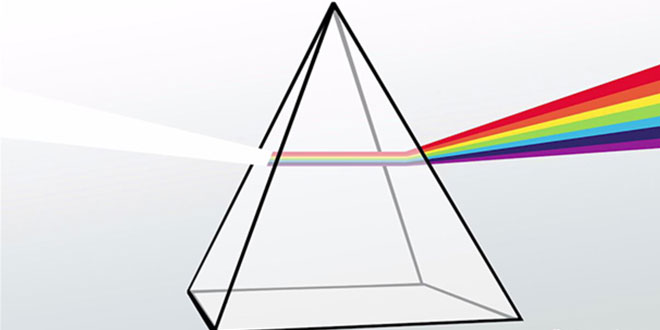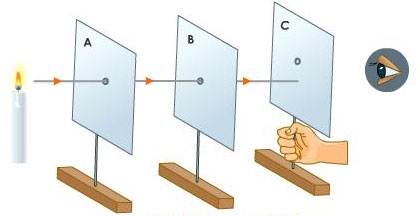Tuesday , July 5 2022# NCERT 7th Class (CBSE) Science: Light

#### Question: What is the difference between a real image and a virtual image? Give one example of each type of image.

1. Real image:The image which can be obtained on a screen is called a real image. In a cinema hall, we see the images of actors and actresses on the screen. So, the images formed on a cinema screen are real images.
2. Virtual image: The images which cannot be obtained on a screen is called a virtual image. A virtual image can be seen only by looking into a mirror or a lens. The image of our face formed by a plane mirror cannot be obtained on a screen, it can be seen only by looking into the mirror. So, the image of our face in a plane mirror is a virtual image.

#### Question: What is the principle axis of a concave mirror?

Answer: A straight line passing through the two centers of curvature of the lens is called the principal axis of the lens.

#### Question: What is principal focus of a convex lens?

Answer: A parallel beam of light passing through a convex lens converges at a single point. This point is called the principal focus (F) of the convex lens. The distance between the principal focus and the optical centre of the convex lens is called the focal length of the lens.

#### Question: Mention the characteristics of an image formed by a plane mirror.

Answer: Characteristics of an image formed by a plane mirror are:

1. The image formed by a plane mirror is always virtual and erect.
2. The image undergoes lateral inversion.
3. The size of the image formed by a plane mirror is the same as that of the object.
4. The distance of the object from the mirror is equal to the distance of the image formed by the mirror (that is, image distance = object distance)

#### Question: With the help of an experiment. Show that light cannot bend from its path.

Answer: To demonstrate that light travels in a straight line or cannot bend from its path, we require three small cardboard sheets, three stands, candle and candle stand.

1. Now make small holes at the centre of the three cardboard sheets.
2. Fix the cardboard sheets on the three stands and arrange them in a straight line.
3. Light the candle and place it in front of the hole made on the first cardboard sheet.
4. See the candle flame through the hole of the third cardboard sheet.
5. Now, move the third cardboard stand a little away from the line.
6. We will observe that candle flame is visible when holes of all the cardboard sheets are in straight line. The light is not visible when third stand is moved away. This shows that light travels in a straight line.
7.## Sources of Energy: 10th Science Chapter 14

Class: 10th Class Subject: Science Chapter: Chapter 14: Sources of Energy Quiz: – Questions MCQs: …

### One comment

1.Please send me the sample papers of Class 7 Science.